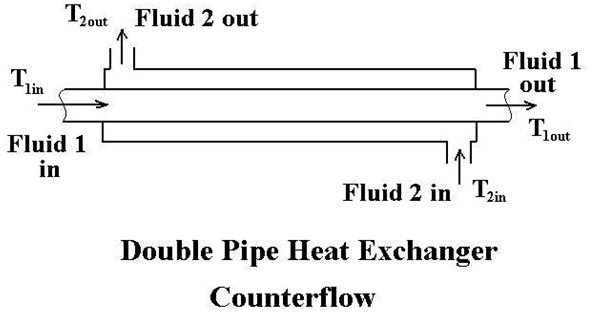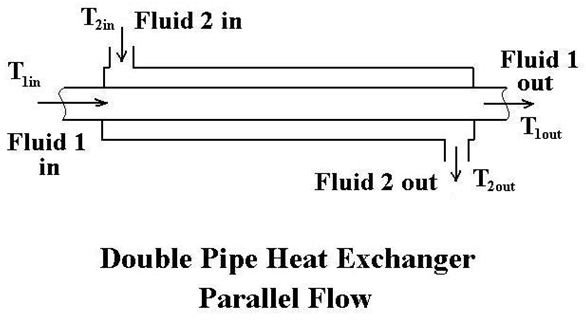# Double Pipe Heat Exchanger Design with Counterflow or Parallel Flow

Page content

## Introduction

In double pipe heat exchanger design, an important factor is the type of flow pattern in the heat exchanger. A double pipe heat exchanger will typically be either counterflow or parallel flow. Crossflow just doesn’t work for a double pipe heat exchanger. The flow pattern and the required heat exchange duty allows calculation of the log mean temperature difference. That together with an estimated overall heat transfer coefficient allows calculation of the required heat transfer surface area. Then pipe sizes, pipe lengths and number of bends can be determined.

## General Configuration and Characteristics of a Double Pipe Heat Exchanger

A double pipe heat exchanger, in its simplest form is just one pipe inside another larger pipe. One fluid flows through the inside pipe and the other flows through the annulus between the two pipes. The wall of the inner pipe is the heat transfer surface. The pipes are usually doubled back multiple times as shown in the diagram at the left, in order to make the overall unit more compact.

The term ‘hairpin heat exchanger’ is also used for a heat exchanger of the configuration in the diagram. A hairpin heat exchanger may have only one inside pipe, or it may have multiple inside tubes, but it will always have the doubling back feature shown. . Some heat exchanger manufacturers advertise the availability of finned tubes in a hairpin or double pipe heat exchanger. These would always be longitudinal fins, rather than the more common radial fins used in a crossflow finned tube heat exchanger.

## Counterflow and Parallel Flow in a Double Pipe Heat ExchangerA primary advantage of a hairpin or double pipe heat exchanger is that it can be operated in a true counterflow pattern, which is the most efficient flow pattern. That is, it will give the highest overall heat transfer coefficient for the double pipe heat exchanger design.Also, hairpin and double pipe heat exchangers can handle high pressures and temperatures well. When they are operating in true counterflow, they can operate with a temperature cross, that is, where the cold side outlet temperature is higher than the hot side outlet temperature.

For example, in the diagrams in this section, consider Fluid 1 to be the hot fluid and Fluid 2 to be the cold fluid. Then, in the counterflow diagram at the left, you can see that the cold side outlet temperature, T2out, can approach the hot side entering temperature, T1in, which is higher than the hot side outlet temperature, T2out. For the parallel flow shown at the right, T2out can only approach T1out; it could not be greater.

## Double Pipe Heat Exchanger Design

Determination of the heat transfer surface area needed for a double pipe heat exchanger design can be done using the basic heat exchanger equation: Q = UA ΔTlm, where:

Q is the rate of heat transfer between the two fluids in the heat exchanger in Btu/hr,

U is the overall heat transfer coefficient in BTU/hr-ft2-oF,

A is the heat transfer surface area in ft2, and

ΔTlm is the log mean temperature difference in oF, calculated from the inlet and outlet temperatures of both fluids.

These parameters in the basic heat exchanger equation are discussed in ‘Fundamentals of Heat Exchanger Design’, and they are used in an example in ‘Preliminary Heat Exchanger Design Example’. After determination of the required heat transfer surface area, the diameter and length of the inner pipe can be selected and then the diameter of the outer pipe. Finally, the length of the straight sections and the number of bends can be selected.

## Image Credit

Double Pipe Heat Exchanger picture: https://doublepipeheatexchanger.com/